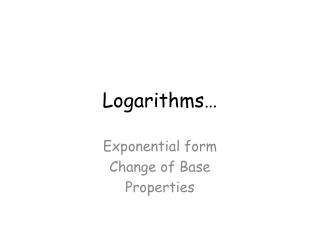DownloadDownload PresentationLogarithms…

# Logarithms…

Télécharger la présentation## Logarithms…

- - - - - - - - - - - - - - - - - - - - - - - - - - - E N D - - - - - - - - - - - - - - - - - - - - - - - - - - -
##### Presentation Transcript

1. Logarithms… Exponential form Change of Base Properties

2. Use your calculator to solve the following • 10x = 32 x = _____ • 10x = 50 x = _____ • 10x = 207 x = _____ • 10x = 2 x = _____

3. Using the Log key on your calculator find each of the following • Log 32= _____ • Log 50= _____ • Log 207 = ____ • Log 2 = _____

4. NO CALCULATOR • If 101.681 = 48, what is log 48 ? _____ • If log 156 = 2.193, what is 102.193 ? __ • Log(103) = ___ • 10log 100 = ____

5. Use your calculator to solve the following • 2x = 32 x = ____ • 2x = 50 x = ____ • 2x = ¼ x = ____ • Log2 ¼ = ___ • Log250 = ___ • Log232 = ___

6. General Form • Logarithmic form • Logba = c • Exponential form • bc = a

7. No Calculator Log864 = ____ Log464 = ____ Log264 = ____ Log6464 = ____ Log81 = ____ Log8 (1/64) = ____

8. No Calculator • If 52.892 = 105, then log5105 = ____ • If log217 = 4.088, what is 24.088 ? • log5(54) = ____ • = ____

9. Natural Log (ln) is a special log with a base e. • Basic Log Properties: • ln 1 and log 1 = 0 • lne = 1 • logbb = 1 • elnx = x • blogbx = x

10. Rules for Logs

11. Examples: Use the properties of logs to expand. Write as a single log:

12. Change of Base • Since our calculators can only evaluate a base of 10 or e, we will let a = 10 or use ln. • This formula allows us to evaluate logs with ANY base using the calculator!

13. Examples Evaluate using change of base: